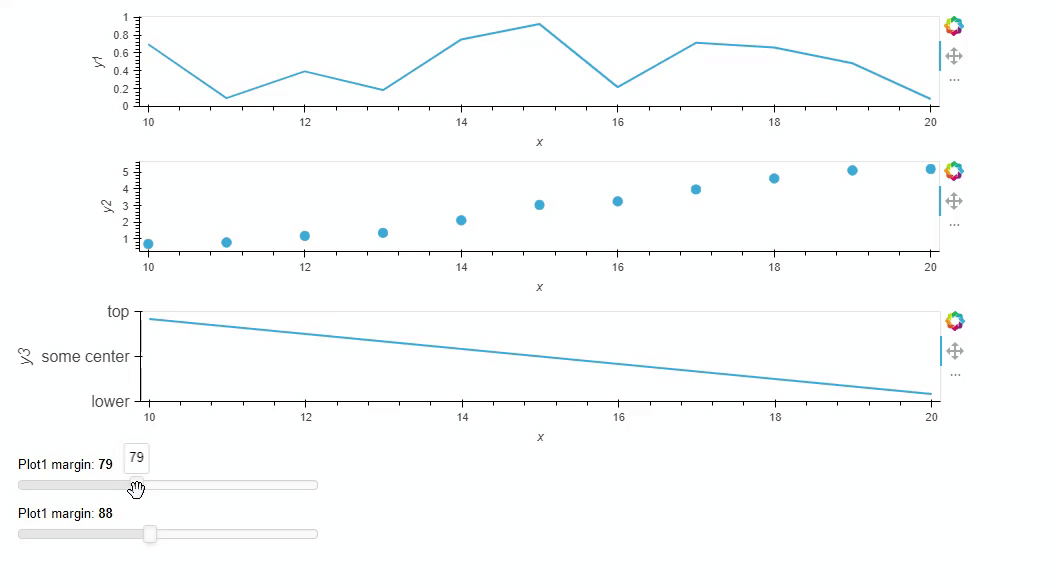# Is there a concept of frame alignment (top, bottom, left, right) in holoviews using bokeh backend?

Sometimes I come across the need to ensure alignment of holoviews plots in a layout based on where the frames either start or end in horizontal or vertical direction. I am not sure if this question is really a bokeh question or holoviews question, but try here first. It could be situations when you got two or more plots and the width of the formatted label ticks are quite different. Then sometimes the plots organized in a column layout might not align for xaxis based on where the frame starts from the left side.
I was wondering if there is a concept of frame alignment (top, bottom, left, right) in holoviews using bokeh backend? I must say I really love the ‘frame_height’ and ‘frame_width’ as opposed to only having ‘height’ and ‘width’ as arguments to elements. These provide ways to have the output much more as one expect it. So hoping there is also something similar for frame aligment.

Here comes a constructed example trying to illustrate the point.

``````# Imports
import pandas as pd
import numpy as np
import holoviews as hv
from holoviews import opts

hv.extension('bokeh')

# Create dummy data
xs = np.linspace(10,20, 11)
y1 = np.random.random(len(xs)) # .cumsum()
y2 = y.cumsum()
y3 = -xs**1/3

data = {
'x': xs,
'y1': y1,
'y2': y2,
'y3': y3
}

df = pd.DataFrame(data)

size_opts = dict(frame_width=800, frame_height=90)
(
hv.Curve(df, kdims='x', vdims='y1')
+ hv.Scatter(df, 'x', 'y2').opts(size=9)
+ hv.Curve(df, 'x', 'y3').opts(yticks=[(-7,'lower'), (-5, 'some center'), (-3, 'top')], fontsize={'ylabel': '12pt', 'yticks': '12pt'})
).cols(1).opts(opts.Curve(**size_opts), opts.Scatter(**size_opts))
``````

Output as beeing plotted in a notebook:

Question is if there is any way to say something like frame_align=‘left’ (or ‘top’, ‘bottom’, ‘right’)
For the plot above a possible solution is to put yaxis to the right and then the left of the plots will typically align, but maybe there is some other better way?

UPDATE: THERE PROPER SOLUTION IS IN A POST BELOW.

Hi @geoviz

I don’t know of a concept. A workaround might be to use Panel to lay out the plots. With Panel you can adjust the margin etc. manually. You can even use widgets to speed up the process of find the right values.

Below is an interactive example.``````# Imports
import pandas as pd
import numpy as np
import holoviews as hv
from holoviews import opts

hv.extension('bokeh')

# Create dummy data
xs = np.linspace(10,20, 11)
y1 = np.random.random(len(xs)) # .cumsum()
y2 = y1.cumsum()
y3 = -xs**1/3

data = {
'x': xs,
'y1': y1,
'y2': y2,
'y3': y3
}

df = pd.DataFrame(data)

size_opts = dict(frame_width=800, frame_height=90)

plot1 = hv.Curve(df, kdims='x', vdims='y1').options(**size_opts)
plot2 = hv.Scatter(df, 'x', 'y2').opts(size=9).options(**size_opts)
plot3 = hv.Curve(df, 'x', 'y3').opts(yticks=[(-7,'lower'), (-5, 'some center'), (-3, 'top')], fontsize={'ylabel': '12pt', 'yticks': '12pt'}).options(**size_opts)

import panel as pn

pn.extension()

M1 =77
M2 = 87
plot1_panel = pn.panel(plot1, margin=(0,0,0,M1))
plot2_panel = pn.panel(plot2, margin=(0,0,0,M2))

layout = pn.Column(
plot1_panel,
plot2_panel,
plot3,
sizing_mode="stretch_width",
)

plot1_margin = pn.widgets.IntSlider(value=M1, start=0, end=200, name="Plot1 margin", sizing_mode="fixed")
plot2_margin = pn.widgets.IntSlider(value=M2, start=0, end=200, name="Plot1 margin", sizing_mode="fixed")

@pn.depends(plot1_margin, watch=True)
def update_margin1(value):
plot1_panel.margin=(0,0,0,value)

@pn.depends(plot2_margin, watch=True)
def update_margin2(value):
plot2_panel.margin=(0,0,0,value)

layout.append(plot1_margin)
layout.append(plot2_margin)
layout.servable()
``````
``````panel serve script.py
``````

Actually. With Panel you can `align` elements.

``````# Imports
import pandas as pd
import numpy as np
import holoviews as hv
from holoviews import opts

hv.extension('bokeh')

# Create dummy data
xs = np.linspace(10,20, 11)
y1 = np.random.random(len(xs)) # .cumsum()
y2 = y1.cumsum()
y3 = -xs**1/3

data = {
'x': xs,
'y1': y1,
'y2': y2,
'y3': y3
}

df = pd.DataFrame(data)

size_opts = dict(frame_width=800, frame_height=90)

plot1 = hv.Curve(df, kdims='x', vdims='y1').options(**size_opts)
plot2 = hv.Scatter(df, 'x', 'y2').opts(size=9).options(**size_opts)
plot3 = hv.Curve(df, 'x', 'y3').opts(yticks=[(-7,'lower'), (-5, 'some center'), (-3, 'top')], fontsize={'ylabel': '12pt', 'yticks': '12pt'}).options(**size_opts)

import panel as pn

pn.extension()

layout = pn.Column(
pn.panel(plot1, align="end"),
pn.panel(plot2, align="end"),
pn.panel(plot3, align="end"),
sizing_mode="stretch_width",
)
layout.servable()
``````

Thanks a lot @Marc for these nice methods to solve the problem. I kind of new about the panel align option, but not that you could define margins so precisely. Great options and good learning!

I still wonder if frame alignment has possibly been discussed for holoviews itself as one could kind of expect that at least axes where the axis values, dimensions and ranges are the same would be possible to align. It is to be said that most often this is not a problem and the plots do align very well out of the box . It is more when label formatting and lengths of labels/presence of labels vary that one could need and benefit from options such as ‘frame_align’ = ‘left’. I believe it could be a great feature in holoviews, but would not know how hard it would be to develop or if it possibly needs development on the bokeh side too…

1 Like